e c m

effective (potential derived) charges for macromolecules in solvent

version 2.00

EMBL, Heidelberg

Mon Jan 19 11:11:11 MET 1998

R.R. Gabdoulline & R.C. Wade. Effective charges for macromolecules in solvent
J.Phys.Chem. (1996) 100, 3868-3878

Abstract
In order to study protein-protein and protein-DNA association, the electrostatic forces and interaction free energies for two macromolecules at different mutual orientations and separations need to be evaluated. Realistic systems typically consist of thousands of atomic charges in an environment with a non-uniform dielectric permittivity and a solvent of non-zero ionic strength. Consequently, accurate evaluations of electrostatic forces and energies for such systems are only computationally feasible for a limited number of macromolecule positions. Here, we show that, by representing each molecule by a small number of effective charges in a uniform dielectric, intermolecular electrostatic interactions can be calculated simply and with high accuracy. The effective charges are derived by fitting them to reproduce the molecular electrostatic potential calculated by numerical solution of the finite-difference linearized Poisson-Boltzmann equation. The derived charges are expected to be useful in applications such as the simulation of the diffusional encounter of proteins where they will provide improved accuracy over the commonly-used test charge approximation.

ECM is not provided alone, but with the SDA software.

• Effective charges for macromolecules in solvent: what they are and when to use them:
• They are fitted charges that, when immersed in a uniform dielectric, give the electrostatic potential of the molecule computed in a heterogeneous dielectric. They can reproduce the electrostatic potential of a protein computed taking account of all atomic charges with an accuracy of 5-10% when only assigning effective charges to 1 or 2 atoms of the side-chains of the titratable residues.
• The fitting is similar to that used in the CHELP procedure to obtain atomic charges for a small molecules: it is done over a layer of reasonable thickness around the molecule (a molecular skin).
• As in finite difference calculations, effective charges can be used to compute interaction energies and forces between two macromolecules taking account of complex molecular shapes, a low dielectric in the molecular interiors and ionic solvent surrounding the molecules. They use the results of finite difference electrostatic potential calculations on each of the molecules when they are separated. The advantage of the effective charges is that the computations can be performed much more quickly. E.g. force calculations in a Brownian dynamics simulation have the same computational cost with effective charges as test charges.
• They not only offer a fast evaluation of the energy, but are also free of the grid spacing problems that are encountered in finite difference methods: the fitting results are not sensitive to the grid spacing used to compute potentials within the error estimate mentioned above.
• Model cases:
• dipole (2 charges of opposite sign at close separation) at the center of low dielectric sphere surrounded by high dielectric gets rescaled by a factor of 1.5;
• a charge at the center of non ionic sphere of radius a surrounded by ionic solvent with Debye-Hückel parameter k gets rescaled by a factor of exp(ka)/(1+ka).

• How to generate effective charges:

BEFORE using the programs the user should have 2 files and know 2 parameters:

• pdb_file - the coordinates of the macromolecule used to compute the electrostatic potential in Brookhaven protein databank format;
• grd_file electrostatic potential grid in UHBD format computed for the macromolecule in exactly the same orientation and position;
• ionic strength of the solvent,
• dielectric permittivity of the solvent.

Version 2.00 notes.
Version 2 allows the charges to be fitted for an angular region of the potential. The user needs to specify coordinates of 2 points (x1dir(3) and x2dir(3)) and the restriction angle (angleg, in grad) in the file "angular.restriction". Then any points forming an angle larger than angleg to the vector (x2dir-x1dir) are omitted from the fitting procedure. Note, that the order of the 2 points in this input file makes a difference. If there are no data in the file "angular.restriction", the fitting procedure does not perform angular restriction.

There are 4 programs for effective charge generation:

I. ecm_mksites (single precision)

usage: ecm_mksites < pdb_file > test_charge_file

reads in pdb file (standard input), locates the sites for effective charges, assigns them the test charge value and writes to standard output in PDB format with charges given in the last column.

comment: OE* OD* NZ NE* atoms of Glu, Asp, Lys, Arg, and N & O* of NTRM and CTRM terminal residues are coded in the current program. The user may edit the output file manually to delete unnecessary or add other relevant sites. Another possibility is to edit subroutine chdef() to allow another automatic selection.

II. ecm_expand (single precision)

usage: ecm_expand < input_script

Expands the electrostatic potential of a macromolecule as given by a set of effective charges in uniform solvent. The resultant effective charges are given in the last column of a PDB format file output in "echa_file". In addition, the matrix for Coulomb-Coulomb, Coulomb-grid, grid-grid overlaps is written in file "matrix". (This is the most expensive part to compute.) The file "matrix" will be used by the subsequent regularization programs under this name. Standard output contains information about the performance of ecm_expand. This includes a simple graphics display of a cross-section of the region over which the potential fitting is performed. The user should check that this bears some resemblance to the shape of the protein under study.

comment: The input script contains the names for: the pdb file, the grid file, the charge sites file generated by ecm_mksites, the name for a file to write effective charges in. An example input file is in file "inp_expand".

comment: The standard version uses a maximal grid size of 110x110x110, a maximal number of charge sites of 180, and a maximal number of fitting points of 70000. Change these values in the first 3 lines of the program if you need more.

comment: The program uses LINPACK routine dgesl() to solve the linear system of equations.

advice: If the fitting results are satisfactory, don't go further. However, save the file "matrix" if you want to probe different regularizations later.

You can check the assignments by reading the output file into a graphics program and colouring the atom types by temperature factor, which is effective charge in this file

III. ecm_regularize (double precision)

usage: ecm_regularize (automatically reads from file "matrix")

Prints out the properties of the matrix equation for expansion. Namely, it exhaustively probes regularization of the matrix equation in the fitting problem equating the last N principal components of charges to the values derived from test charges. The output gives the N-dependence of the deviations (RMSD) of the effective charge values from those of the test charges, the fitting error, the maximum deviation of any one effective charge from the respective test charge value (RM1D), the square root of the fitting error, and the sum of all the effective charges.

comment: The program is optional, in that it just helps the user to choose N - the level of regularization, which should give small enough deviations and small enough fitting errors. The optimization is not necessary if the derived charges are to be used only in the fitting region - the smaller the fitting errors are the better. Usually one needs to use the charges in other regions without introducing artifacts - then it is necessary to have effective charges close to some realistic numbers - therefore the need to minimize the deviation from the test charge values which give a reasonable description of close-range interactions.

comment: The program uses EISPACK routine rs() to perform matrix diagonalization.

definition: RMSD =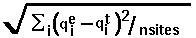, where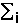means summation over i=1,...,nsites,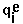- effective charge on site i,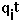- test charge on site i.

definition: RM1D =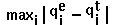.

definition: fitting_error=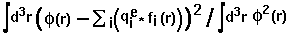, where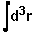means integration over fitting region,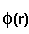- electrostatic potential of a macromolecule,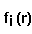- Coulomb-Debye potential of a unit charge in a uniform solvent. One should mentally take a square root from this in order to imagine errors in the magnitude of the fitting potential, even though the definition is standard.

IV. ecm_mkecharges (double precision)

usage: ecm_mkecharges (automatically uses file "matrix")

writes regularized effective charges to a file "echa_file_R". An old format file with charges scaled by the solvent dielectric constant is written to "echa_file_E". The only input the program requires is the integer number, N - the regularization level.

comment: The program uses EISPACK routine rs() to perform matrix diagonalization.

Usage example

• make sure files "1brsA.pdb", "1brsA.grd" and "inp_expandS" are present
• type ecm_mksites < 1brsA.pdb > 1brsA.tchaS
• type ecm_expand < inp_expandS
• if charges in file "1brsA.echaS" and reported errors look OK, then use these charges to represent the potential of the macromolecule "1brsA.pdb"
• otherwise type ecm_regularize and inspect the output table to choose an appropriate value of N. Then
• type ecm_mkecharges and, at the prompt, type the value of N chosen to get "1brsA.echaS_R" - regularized at the level of N charges.
• if you don't like the results - try the previous step again with another value of N.

Remark. The figure below left shows the meaning of optimization when using regularized charges. In this example, 47 charge sites were used to fit the potential over distances 5-8 Angstroms from the van der Waals surface of the macromolecule in file "1brsA.pdb". The maximum accuracy is obtained without regularization (N=0) when the standard classical error is 0.037. This corresponds to an average error of 19% in the computed potential values. For test charges (N=47), the standard error is 0.31 corresponding to an error of 56% in the potential. However, the most accurate effective charges deviate from "reasonable" test charge values (+-0.5,1.0) by 3.5 units on average. Examination of the error curve shows that if the regularization level is chosen at N=20, the error in the potential is only 23%, and the charges deviate from test charge values by an average of only 0.6 units (and have a maximal deviation (RM1D) of 1.9 - this is seen from the output of ecm_regularize). Better accuracy of 20% is given by N=10, with an RMSD for effective charges of 1.2 and an RM1D of 3.2. Poorer accuracy of 29% is given by N=30, with an RMSD for effective charges of 0.4 on average and an RM1D of 0.8. Automated optimization is difficult even in this case: the error increase with optimization level allows at least the above 3 optimum choices. The use of other sites would mean that this curve has another structure.
An example is given in the figure below right where 92 charge sites are used. Here, regularization at level 40 allows approximation of the potential within an error of 14% with RMSD deviation of charges 0.55 (RM1D - 1.6). The smallest fit error is 10%, compared to 20 in previous case.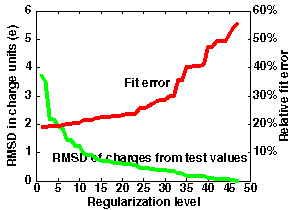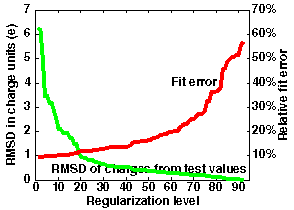An example of usage is given in the directory example where all the fitting and regularization is done by one script doal .

Viel Spass.

Razif Gabdoulline

Mon Jan 19 11:11:11 MET 1998

Imprint/Privacy